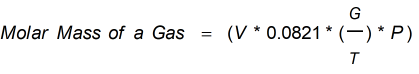Request a Tool

Molar Mass Calculator

Mass of a sample of that compound divided by the amount of substance

Output

Molar Mass of a Gas
0

Formula• V = Volume
• M = Mass of Gas
• T = Temperature
• P = Pressure

Defination / Uses

The mass of a sample of a chemical compound divided by the amount of substance in that sample, defined in moles, is the molar mass of that compound.

molar mass calculator tool will help you in calculating the molar mass of a material. The mass of a sample of a chemical compound divided by the amount of material in that sample, measured in moles, is the molar mass of that compound in chemistry. The molar mass of a substance is a bulk attribute, not a molecular property. The molar mass is a weighted average of many different instances of the compound, which can vary in mass due to isotopes. The molar mass is most usually calculated from standard atomic weights and is thus a terrestrial average and a function of the relative abundance of the constituent atoms on Earth. For bulk quantities, the molar mass is ideal for converting between the mass of a substance and the amount of a substance. Use the upper given formula for manual calculations. No sign-up, registration OR captcha is required to use this tool.